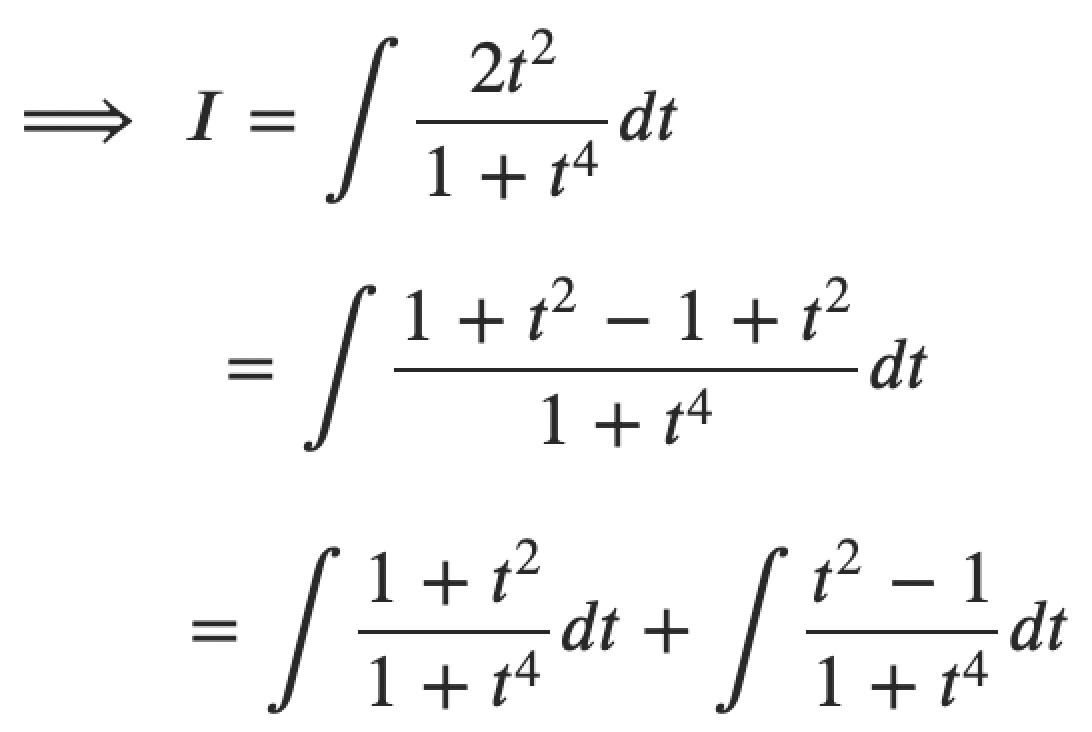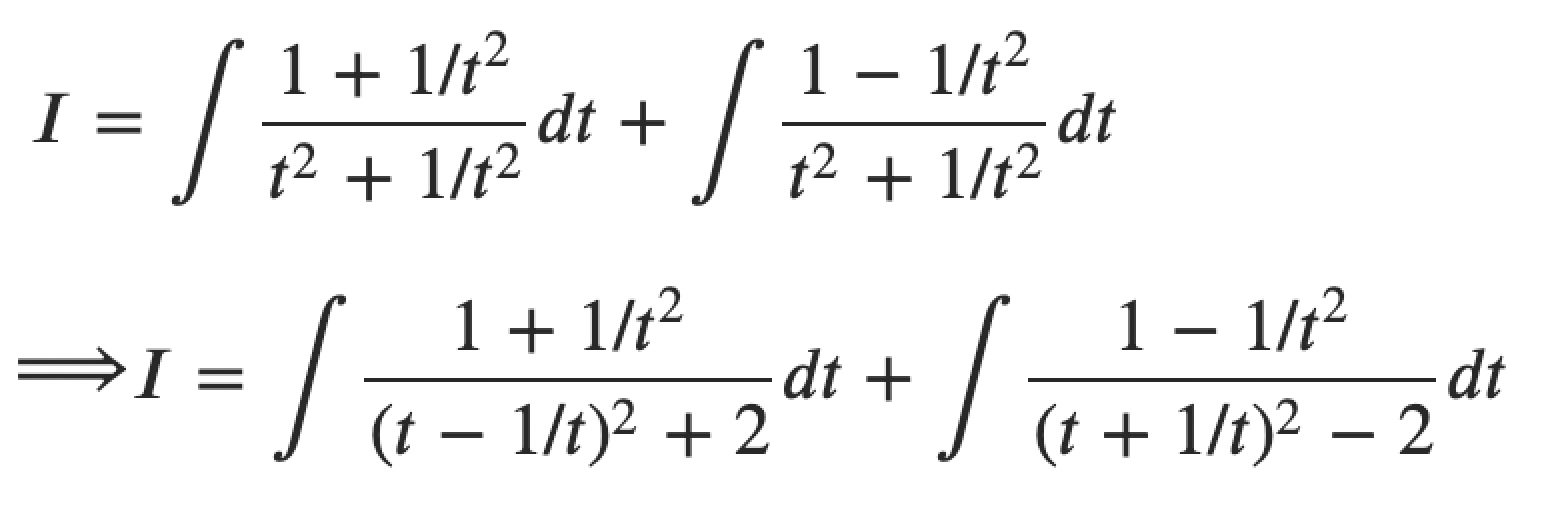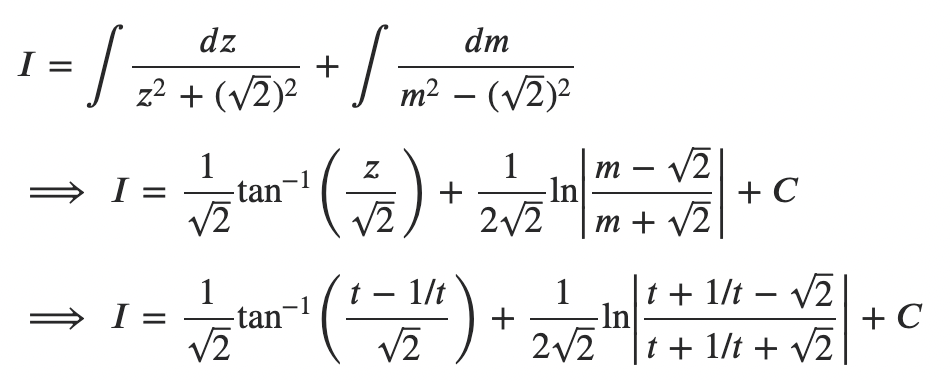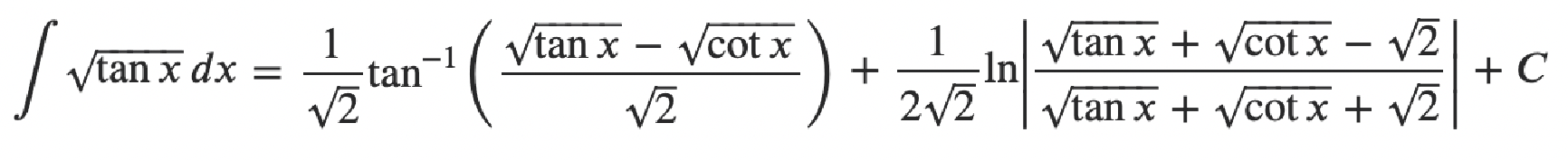# Integration of Tan x

Integration of tan x is equivalent to log |sec x| + C.

Integration of Tan x means finding the integral of the trigonometric function tan x. The integral of tan x with respect to x can be written as ∫ tan x dx. Here, we need to find the indefinite integral of tan x. So, the integration of tan x results in a new function and an arbitrary constant C. Let’s know the formula for the integration of tan x, along with the derivation and examples.

## Integration of Tan x Formula

The formula for the integration of tan x into dx is given by:

 ∫ tan x dx = log |sec x| + C

Or

 ∫ tan x dx = -log |cos x| + C

## Integration of Tan x dx Derivation

∫ tan x dx

We know that tan A = sin A/cos A

Thus, ∫ tan x dx = ∫ (sin x /cos x) dx

= ∫ (1/cos x) sin x dx

Let’s apply the substitution method of integration.

Let t = cos x

⇒ dt = – sin x dx

⇒ sin x dx = -dt

So, ∫ tan x dx = ∫(sin x /cos x) dx = ∫ (1/t) (-dt) = – ∫ (1/t) dt

– log |t| + C

= -log |cos x| + C

= log |(cos x)-1| + C

= log |1/cos x| + C

= log |sec x| + C

Therefore, ∫ tan x dx = -log |cos x| + C = log |sec x| + C

### Examples on Integration of Tan x

Example 1: Integration of Tan x whole square

Integral of tan x whole square can be written as:

∫ (tan x)2

Let us find the integral of (tan x)2 with respect to dx.

∫ (tan x)2 dx = ∫ tan2x dx

Using the identity sec2A – tan2A = 1,

∫ tan2x dx = ∫ (sec2x – 1) dx

= ∫ sec2x dx – ∫ 1 dx

Using the standard integration formulas, ∫sec2x dx = tan x + C and ∫ 1 dx = x + C, we get;

= tan x – x + C

Therefore, ∫ (tan x)2 dx = (tan x) – x + C

Example 2: Integration of under root tan x

Integral of under root tan x can be written as:

∫ √(tan x)

Let’s find the integral of under root tan x with respect to x.

∫ √(tan x) dx

Let tan x = t2 such that tan2x = t4 and sec2x = 1 + t4.

Differentiate with respect to x.

⇒ sec2x dx = 2t dt

⇒ dx = [2t / (1 + t4)]dtDividing the numerator and denominator of RHS by t2, we get;Let us substitute t + 1/t = m and t – 1/t = z.

Also, by differentiating these equations with respect to t, we get;

1 – 1/t2 = dm/dt and 1 + 1/t2 = dz/dt

⇒ (1 – 1/t2) dt = dm and (1 + 1/t2) dt = dzNow, substitute t = √(tan x) and 1/t = 1/√(tan x) = √(cot x).